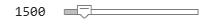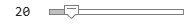## Choose Between `spmd`, `parfor`, and `parfeval`

### Communicating Parallel Code

To run computations in parallel, you can use `parfor`, `parfeval`, `parfevalOnAll`, or `spmd`. Each construct relies on different parallel programming concepts. If you require workers to communicate throughout a computation, use `parfeval`, `parfevalOnAll`, or `spmd`.

• Use `parfeval` or `parfevalOnAll` if your code can be split into a set of tasks, where each task can depend on the output of other tasks.

• Use `spmd` if you require communication between workers during a computation.

Computations with `parfeval` are best represented as a graph, similar to a Kanban board with blocking. Generally, results are collected from workers after a computation is complete. You can collect results from execution of a `parfeval` operation by using `afterEach` or `afterAll`. You typically use the results in further calculations.

Computations with `spmd` are best represented by a flowchart, similar to a waterfall workflow. A pool worker executing `spmd` statements is called a lab. Results can be collected from labs during a computation. Sometimes, labs must communicate with other labs before they can finish their computation.

If you are unsure, ask yourself the following: within my communicating parallel code, can each computation be completed without any communication between workers? If yes, use `parfeval`. Otherwise, use `spmd`.

#### Synchronous and Asynchronous Work

When choosing between `parfor`, `parfeval`, and `spmd`, consider whether your calculation requires synchronization with the client.

`parfor` and `spmd` require synchronization, and therefore block you from running any new computations on the MATLAB® client. `parfeval` does not require synchronization, so the client is free to pursue other work.

### Compare Performance of Multithreading and `ProcessPool`

In this example, you compare how fast functions run on the client and on a `ProcessPool`. Some MATLAB functions make use of multithreading. Tasks that use these functions perform better on multiple threads than a single thread. Therefore, if you use these functions on a machine with many cores, a local cluster can perform worse than multithreading on the client.

The supporting function `clientFasterThanPool`, listed at the end of this example, returns `true` if multiple executions are performed faster on the client than a `parfor`-loop. The syntax is the same as `parfeval`: use a function handle as the first argument, the number of outputs as the second argument, and then give all required arguments for the function.

First, create a local `ProcessPool`.

`p = parpool('local');`
```Starting parallel pool (parpool) using the 'local' profile ... Connected to the parallel pool (number of workers: 6). ```

Check how fast the `eig` function runs by using the `clientFasterThanPool` supporting function. Create an anonymous function with `eig` to represent your function call.

`[~, t_client, t_pool] = clientFasterThanPool(@(N) eig(randn(N)), 0, 500)`
```t_client = 22.6243 ```
```t_pool = 4.9334 ```

The parallel pool computes the answer faster than the client. Divide `t_client` by `maxNumCompThreads` to find the time taken per thread on the client.

`t_client/maxNumCompThreads`
```ans = 3.7707 ```

Workers are single threaded by default. The result indicates that the time taken per thread is similar on both the client and the pool, as the value of `t_pool` is roughly 1.5 times the value of `t_client/maxNumCompThreads`. The `eig` function does not benefit from multithreading.

Next, check how fast the `lu` function runs by using the `clientFasterThanPool` supporting function.

`[~, t_client, t_pool] = clientFasterThanPool(@(N) lu(randn(N)), 0, 500)`
```t_client = 1.0225 ```
```t_pool = 0.4693 ```

The parallel pool typically computes the answer faster than the client if your local machine has four or more cores. Divide `t_client` by `maxNumCompThreads` to find the time taken per thread.

`t_client/maxNumCompThreads`
```ans = 0.1704 ```

This result indicates that the time taken per thread is much less on the client than the pool, as the value of `t_pool` is roughly 3 times the value of `t_client/maxNumCompThreads`. Each thread is used for less computational time, indicating that `lu` uses multithreading.

Define Helper Function

The supporting function `clientFasterThanPool` checks whether a computation is faster on the client than on a parallel pool. It takes as input a function handle `fcn` and a variable number of input arguments (`in1, in2, ...`). `clientFasterThanPool` executes `fcn(in1, in2, ...)` on both the client and the active parallel pool. As an example, if you wish to test `rand(500)`, your function handle must be in the following form:

`fcn = @(N) rand(N);`

Then, use `clientFasterThanPool(fcn,500)`.

```function [result, t_multi, t_single] = clientFasterThanPool(fcn,numout,varargin) % Preallocate cell array for outputs outputs = cell(numout); % Client tic for i = 1:200 if numout == 0 fcn(varargin{:}); else [outputs{1:numout}] = fcn(varargin{:}); end end t_multi = toc; % Parallel pool vararginC = parallel.pool.Constant(varargin); tic parfor i = 1:200 % Preallocate cell array for outputs outputs = cell(numout); if numout == 0 fcn(vararginC.Value{:}); else [outputs{1:numout}] = fcn(vararginC.Value{:}); end end t_single = toc; % If multhreading is quicker, return true result = t_single > t_multi; end```

### Compare Performance of `parfor`, `parfeval`, and `spmd`

Using `spmd` can be slower or faster than using `parfor`-loops or `parfeval`, depending on the type of computation. Overhead affects the relative performance of `parfor`-loops, `parfeval`, and `spmd`.

For a set of tasks, `parfor` and `parfeval` typically perform better than `spmd` under these conditions.

• The computational time taken per task is not deterministic.

• The computational time taken per task is not uniform.

• The data returned from each task is small.

Use `parfeval` when:

• You want to run computations in the background.

In this example, you examine the speed at which matrix operations can be performed when using a `parfor`-loop, `parfeval`, and `spmd`.

First, create a local parallel pool `p`.

`p = parpool('local');`
```Starting parallel pool (parpool) using the 'local' profile ... Connected to the parallel pool (number of workers: 6). ```

Compute Random Matrices

Examine the speed at which random matrices can be generated by using a `parfor`-loop, `parfeval`, and `spmd`. Set the number of trials (`n`) and the matrix size (for an `m`-by-`m` matrix). Increasing the number of trials improves the statistics used in later analysis, but does not affect the calculation itself.

```m =1000; n =20;```

Then, use a `parfor`-loop to execute `rand(m)` once for each worker. Time each of the `n` trials.

```parforTime = zeros(n,1); for i = 1:n tic; mats = cell(1,p.NumWorkers); parfor N = 1:p.NumWorkers mats{N} = rand(m); end parforTime(i) = toc; end```

Next, use `parfeval` to execute `rand(m)` once for each worker. Time each of the `n` trials.

```parfevalTime = zeros(n,1); for i = 1:n tic; f(1:p.NumWorkers) = parallel.FevalFuture; for N = 1:p.NumWorkers f(N) = parfeval(@rand,1,m); end mats = fetchOutputs(f, "UniformOutput", false)'; parfevalTime(i) = toc; clear f end```

Finally, use `spmd` to execute `rand(m)` once for each lab. For details on labs and how to execute commands on them with `spmd`, see Run Single Programs on Multiple Data Sets. Time each of the `n` trials.

```spmdTime = zeros(n,1); for i = 1:n tic; spmd e = rand(m); end eigenvals = {e{:}}; spmdTime(i) = toc; end```

Use `rmoutliers` to remove the outliers from each of the trials. Then, use `boxplot` to compare the times.

```% Hide outliers boxData = rmoutliers([parforTime parfevalTime spmdTime]); % Plot data boxplot(boxData, 'labels',{'parfor','parfeval','spmd'}, 'Symbol','') ylabel('Time (seconds)') title('Make n random matrices (m by m)')```Typically, `spmd` requires more overhead per evaluation than `parfor` or `parfeval`. Therefore, in this case, using a `parfor`-loop or `parfeval` is more efficient.

Compute Sum of Random Matrices

Next, compute the sum of random matrices. You can do this by using a reduction variable with a `parfor`-loop, a sum after computations with `parfeval`, or `gplus` with `spmd`. Again, set the number of trials (`n`) and the matrix size (for an `m`-by-`m` matrix).

```m =1000; n =20;```

Then, use a `parfor`-loop to execute `rand(m)` once for each worker. Compute the sum with a reduction variable. Time each of the `n` trials.

```parforTime = zeros(n,1); for i = 1:n tic; result = 0; parfor N = 1:p.NumWorkers result = result + rand(m); end parforTime(i) = toc; end```

Next, use `parfeval` to execute `rand(m)` once for each worker. Use `fetchOutputs` on all of the matrices, then use `sum`. Time each of the `n` trials.

```parfevalTime = zeros(n,1); for i = 1:n tic; f(1:p.NumWorkers) = parallel.FevalFuture; for N = 1:p.NumWorkers f(N) = parfeval(@rand,1,m); end result = sum(fetchOutputs(f)); parfevalTime(i) = toc; clear f end```

Finally, use `spmd` to execute `rand(m)` once for each lab. Use `gplus` to sum all of the matrices. To send the result only to the first lab, set the optional `targetlab` argument to `1`. Time each of the `n` trials.

```spmdTime = zeros(n,1); for i = 1:n tic; spmd r = gplus(rand(m), 1); end result = r{1}; spmdTime(i) = toc; end```

Use `rmoutliers` to remove the outliers from each of the trials. Then, use `boxplot` to compare the times.

```% Hide outliers boxData = rmoutliers([parforTime parfevalTime spmdTime]); % Plot data boxplot(boxData, 'labels',{'parfor','parfeval','spmd'}, 'Symbol','') ylabel('Time (seconds)') title('Sum of n random matrices (m by m)')```For this calculation, `spmd` is significantly faster than a `parfor`-loop or `parfeval`. When you use reduction variables in a `parfor`-loop, you broadcast the result of each iteration of the `parfor`-loop to all of the workers. By contrast, `spmd` calls `gplus` only once to do a global reduction operation, requiring less overhead. As such, the overhead for the reduction part of the calculation is $O\left({n}^{2}\right)$ for `spmd`, and $O\left(m{n}^{2}\right)$ for `parfor`.